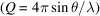International
Tables for
Crystallography
Volume G
Definition and exchange of crystallographic data
Edited by S. R. Hall and B. McMahon

International Tables for Crystallography (2006). Vol. G, ch. 3.3, p. 127

## Section 3.3.8.4. Energy-dispersive X-ray detection

B. H. Tobya*

aNIST Center for Neutron Research, National Institute of Standards and Technology, Gaithersburg, Maryland 20899-8562, USA
Correspondence e-mail: brian.toby@nist.gov

#### 3.3.8.4. Energy-dispersive X-ray detection

| top | pdf |

For energy-dispersive X-ray diffraction, an X-ray detector is placed at a fixed value ofand a diffractogram is measured on a multichannel analyser. The channel number is then calibrated to yield photon energies. From the energy andangle, a d-spacing or Q valueis calculated for each diffraction point. Note that energy, d spacing or Q are not the experimental independent variable. Rather, they result from processing, since calibration information is required. The calibration equation should be described in _pd_calibration_conversion_eqn.

In Example 3.3.8.4, the nominalsetting is 6.5°, but the actual position (determined by prior calibration) is 6.6071°, so the difference is indicated using a _pd_calib_2theta_offset value (see Section 3.3.4.3).

#### Example 3.3.8.4. Measurements from an energy-dispersive X-ray diffraction experiment.